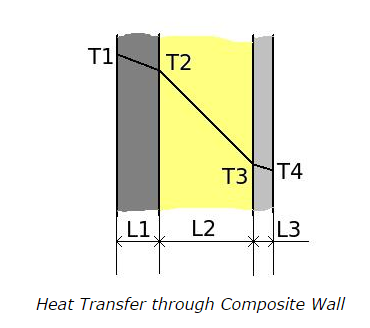Q1. What does a composite wall mean?

a. two walls of different materials are connected in series without any gap between them
b. three walls of different materials are connected in series without any gap between them
c. more than three walls of different materials are connected in series without any gap between them
d. all of the above

ANSWER: d. all of the above

Q2. When a composite wall of three layers in series having thermal resistances R1, R2 and R3 respectively. The heat transfer takes place normal to the surface of the layers. How is the total thermal resistance of the composite system calculated?

a. 1 / (R1 + R2 + R3)
b. ( (1 / R1) + (1 / R2) + (1 / R3) )
c. (R1 + R2 + R3)
d. none of the above

ANSWER: c. (R1 + R2 + R3)

Q3. Calculate the rate of heat flow through a composite wall of having three layers. The thicknesses of three layers are 0.5m, 1m and 0.2m respectively. Thermal conductivity of these layers are 2.5 W/mK, 2 W/mK and 2.2 W/mK respectively. The temperature T1 and T4 are maintained at 1000 K and 200 K respectively. Take surface area of heat transfer 1 m2.a. 1011.5 W/m2
b. 911.5 W/m2
c. 501.5 W/m2
d. 1211.5 W/m2